# Pseudo-Derivative Feedback Controller for Automatic Generation Control in a Deregulated Power System with Hydrogen Energy Storage

Pseudo-Derivative Feedback Controller for Automatic Generation Control in a Deregulated Power System with Hydrogen Energy Storage

G. Ganesan Subramanian T. Suresh Padmanabhan I.A. Chidambaram B. Paramasivam

Department of EEE, E.G.S. Pillay Engineering College (Autonomous), Nagapattinam, Tamil Nadu, India

Department of Electrical Engineering, Annamalai University, Annamalainagar, Tamilnadu, India

Corresponding Author Email:
ganesan@egspec.org; sureshsilver@gmail.com; driacdm@yahoo.com; bpssivam@gmail.com
Page:
84-94
|
DOI:
https://doi.org/10.14447/jnmes.v24i2.a05
6 January 2021
|
Accepted:
22 March 2021
|
Published:
30 June 2021
| Citation
Abstract:

This paper is focused on design and application of Pseudo-Derivative Feedback (PDF) controller for Automatic Generation Control (AGC) of a two-area thermal reheat interconnected power system treated in deregulated condition. The proposed controller gains are tuned simultaneously using Flower Pollination Algorithm (FPA) in order to achieve the optimal transient response of the test system. The control performance of the PDF controller is compared with Proportional Integral (PI) and Proportional Integral Derivative (PID) controllers. Further to improve the AGC performance, Hydrogen Energy Storage (HES) are included in its control area. The execution of HES unit captures the underlying fall in frequency as well as the tie line control power deviations after a sudden load unsettling influence. The simulation results demonstrate that the proposed PDF controller enhance the dynamic response of the deregulated power system as compared with PI and PID contrtoller. The frequency oscillation and tie-line power deviations in the control zones are reduced and the settling time is additionally enhanced when HES unit takes an interest in the frequency regulation along with the traditional generators. Additionally, the Power System Restoration Indices (PSRI) is figured in view of system dynamic performances and the remedial measures to be taken can be decreed. These PSRI shows that the ancillary service requirement to enhances the effectiveness of physical task of the power system with the expanded transmission limit in the system. The presence of an Hydrogen Energy Storage (HES) water electrolyser coupled to a fuel cell improves significantly the control and operation of an energy system and provides good margin of stability of the grid system compared to that a system without HES unit.

Keywords:

automatic generation control, flower pollination algorithm, hydrogen energy storage, PDF controller, power system restoration indices

1. Introduction

The supply of stable and reliable power to the consumer is the primary concern of an interconnected power system framework. Because of the dynamic idea of load, it is important to keep up the harmony between generation and load demand. There by enhancing the execution of the generating unit of frequency and tie line power should be confined in prescribed limit is known as Automatic generation control (AGC).

A critical up-to-date literature review explaining all aspects of AGC is reported in [1–3]. In deregulated environment there are certain market players like generating companies (Gencos), distributing companies (Discos) and transmitting companies (Trancos). This means the power industry is divided into three different sectors and the biding between Discos and Gencos is controlled by the Independent System Operator (ISO). In such a new scenario, Discos can autonomously make agreement with Gencos for delivery power to meet the demand of the consumer. An ISO is a self-governing agent that manages all the transactions alleged between Discos and Gencos. A Disco Participation Matrix (DPM) is used for hallucination of bonds between Gencos and Discos [4, 5]. Regarding few secondary controllers namely, proportional-integral (PI), proportional-integral-derivative (PID) are found in the literature of AGC. These controllers are the first stage closed loop controllers designed for overcome the limitations of open loop control system. The performances for Integral (I), Proportional–Integral (PI), Integral–Derivative (ID), and Proportional–Integral–Derivative (PID) controllers in AGC are practically the same from the viewpoint of dynamic responses . However, the proposed Pseudo-Derivative Feedback controller (PDF) ives much preferred reaction over the previously mentioned controllers. In this study PDF controllers are planned and actualized utilizing FPA calculation in AGC circle of the interconnected restructured power system. The test results demonstrated that the precision and speed execution of the PDF controller had beated the other PI and PID controller.

Many looms such as Particle Swarm Optimization (PSO), Genetic Algorithm (GA), Biogeography-Based Optimization (BBO), Krill Herd Algorithm (KHA), Teaching Learning Based Optimization (TLBO) and Bacterial Foraging Optimization (BFO) algorithm have been planned to resolve the control parameters of a several standard controllers to solve the AGC problem. A wide range of control approachs along with their compensation and boundaries is given in [7-9].

Big Bang Big Crunch (BBBC) is an optimization based on the big bang theory and big crunch hypothesis [10, 11]. From written study, the improvement of power system execution relies upon the control structure as well as on the very much tuned controllers. For this reason, a number of artificial optimization techniques are utilized. So another superior heuristic advancement algorithms are constantly welcome to take care of genuine issues. Flower Pollination Algorithm (FPA) is a newly developed heuristic optimization method based on Pollination of flowers. It has a single key parameter p (switch probability) which makes the calculations simpler to actualize and speedier to achieve ideal arrangement [12, 13]. FPA has uncommon abilities, for example, broad area seek with quality and consistency arrangement .

Balancing of power supply and demand is always a intricate procedure particularly at tip load conditions. As a result, there may be grim alarm about reliable operation of power system. So, it is essential to include Fast-acting Energy Storage Systems (ESS) contains storage capacity in adding up with kinetic energy of the generator rotors is wise to damp out the frequency oscillations [15, 16]. In this study the concept of AGC in two-area thermal power system having a coordinated control action with Hydrogen Energy Storage (HES) units are proficient of controlling the network performance in a very fast manner and to improve power transfer limits for better restoration. In Electrochemical Capacitor or Battery systems have 2 main drawbacks:

• Slow in charging and faster in discharging.

• Residue handling (cleaning) that requires 2 or 3 times a week.

Some have more amount of self discharge compared to HES. Hence, if the HES can be replaced by Electrochemical Capacitor or Battery systems, the results will not give better marginal stability to the system.

The modern power system is pushed near basic working points of confinement in the market condition. High limit and long transmission systems are generally used to take care of the power supply demand of present day society. Huge scale power outage hazards still exist and are inescapable, in spite of the fact that a lot of work has been done to make control on power system versatile against blackouts

An appropriate restoration plan can adequately relieve the negative effect on people in general, the economy, and the power system itself. The targets of restorations are to empower the power framework to come back to ordinary conditions safely and quickly, limit misfortunes and rebuilding time, and reduce unfriendly effects on society.

The goal of power system rebuilding research is to discover quick and dependable approaches to reestablish a power framework to its typical operational state after a black-out event. The motivation behind this paper is to give an adroitly computational philosophy to guaranteeing the system restoration strategies in a faster manner. To accomplish a speedier restoration process, new black start generators can be introduced permitting system reconfigurations and the load recovery can also be adopted in accelerating the system restoration. In this investigation to grow more compelling and quick restoration in the interconnected power system by registering different Power System Restoration Indices (PSRI) for two-area interconnected thermal-hydro and thermal-diesel power system in a restructured domain. From the simulated results it is seen that the restoration procedures for the system with diesel units ensures improved PSRI which provides greater and good margin of stability.

2. Test System for AGC in Restructured Power System

AGC in restructured power system having three conceivable contracts, such as Poolco based transaction, bilateral transactions and hybrid transaction. In Poolco based transaction contract between Gencos and Discos in same area and in the case of bilateral contract any Discos have the freedom to choose any Gencos in their own area or any other control area. Keeping in mind the end goal has to meet the various types of contracts in restructured power system is visualized through disco investment lattice (DPM) . The quantity of lines and sections in a DPM speaks to the quantity of Gencos and Discos separately . Every section in the DPM is noted as Contract Participation Factor (CPF), which speaks to the division of aggregate power shrunk by jth Disco from ith Genco. Thought about first test restructured, deregulated model of a two area thermal-thermal system in which each area consists of two thermal Gencos and two Discos. The relating DPM will progress toward becoming

DPM=\left[ \begin{align} & cp{{f}_{11}}\ \ \ \ \ cp{{f}_{12}}\ \ \ \ \ cp{{f}_{13}}\ \ \ \ \ cp{{f}_{14}} \\ & cp{{f}_{21}}\ \ \ \ \ cp{{f}_{22}}\ \ \ \ \ cp{{f}_{23}}\ \ \ \ \ cp{{f}_{24}}\ \\ & cp{{f}_{31}}\ \ \ \ \ cp{{f}_{32}}\ \ \ \ \ cp{{f}_{33}}\ \ \ \ \ cp{{f}_{34}} \\ & cp{{f}_{41}}\ \ \ \ \ cp{{f}_{42}}\ \ \ \ \ cp{{f}_{43}}\ \ \ \ \ cp{{f}_{44}} \\ \end{align} \right]                   (1)

where cpf represents “Contract Participation Factor” and is like signals that holds the information to which the Genco has to track the load demanded the Disco. The actual and planned steady state power flow via the tie-line are given

\begin{align} & \Delta {{P}_{tie1-2,\ scheduled\ \ \ }}= \\ & \sum\limits_{i=1}^{2}{\sum\limits_{j=3}^{4}{cp{{f}_{ij}}\ }}\Delta {{P}_{L\ j}}\ \ -\ \sum\limits_{i=3}^{4}{\sum\limits_{j=1}^{2}{cp{{f}_{ij}}\ }}\Delta {{P}_{L\ j}} \\ \end{align}                  (2)

$\Delta {{P}_{tie\ 1-2,\ actual\ \ \ }}=\left( \ 2\ \pi \ {{T}_{12}}/\ s\ \right)\ \left( \Delta {{F}_{1}}-\Delta {{F}_{2}} \right)$                     (3)

At some random time, the tie-line power error $\Delta P_{\text {tie } 1-2, \text { error }}$ is defined as

$\Delta {{P}_{tie1-2,\ error\ \ \ }}=\ \Delta {{P}_{tie1-2,\ actual\ \ \ }}-\ \ \Delta {{P}_{tie1-2,\ scheduled\ \ \ }}$             (4)

The error signal is used to cause the respective ACE signals as in the traditional scenario

$AC{{E}_{1}}\ =\ {{\beta }_{1}}\Delta {{F}_{1}}\ +\Delta {{P}_{tie\ 1-2,\ error}}$                 (5)

$AC{{E}_{2}}\ =\ {{\beta }_{2}}\Delta {{F}_{2}}\ +\Delta {{P}_{tie\ 2-1,\ error}}$                        (6)

For two area system as shown in Figure 1, the contracted power supplied by ith Genco is

$\Delta P{{g}_{i}}\ =\ \sum\limits_{j=1}^{DISCO\ =4}{cp{{f}_{ij}}}\Delta {{P}_{L}}_{j}$               (7)

Also note that $\Delta P_{L_{1, L O C}}=\Delta P_{L_{1}}+\Delta P_{L_{2}}, \Delta P_{L_{2, L O C}}=\Delta P_{L_{3}}+\Delta P_{L_{4}}$.

In the proposed AGC usage, the contracted load is bolstered forward through the DPM lattice to Genco set points. The real loads affect system dynamics via the input $\Delta P_{L, L O C}$ to the power system blocks. Any crisscross amongst genuine and contracted demands will result in frequency deviations that will drive LFC to re-dispatch the Gencos according to ACE participation factors i.e., apf11, apf12, apf21 and apf22.

## 1.pngFigure 1. Linearized model of a two-area thermal-thermal interconnected power system in a restructured environment

The proposed PDF controllers are configuration utilizing FPA and implemented two-kinds of test system, for example, two-area thermal-thermal power system and are contrasted with the output responses of the system considered with the PI an PID controllers. The point by point little perturbation exchange transfer function block diagram model of the two-area thermal- themal is shown in Figure 1.

3. Design of PDF Controllers Using FPA

The structure of Pseudo-derivative feedback controller gives all the control parts of PID control, however without system zeros that are typically presented by a PID compensator. The proposed PDF controller, the rate of the deliberate parameter is sustained back without calculating a derivative. The block diagram of PDF controller structure feed's back a proportional component of the measurement rather than the error, following the integrator is demonstrated particle Figure 2. The PDF controller repaid systeem has definitely no overshoot, however the PI system having indistinguishable damping, has overshoot caused by the zero at Ki/Kp. The zero presented by the PI control does anyway give more lead the control, and hence a quicker ascent time, however to the detriment of overshoot . In this examination KP and KI esteem are tuned utilizing Flower Pollination Algorithm (FPA) method. The primary function of AGC is to control load frequency and tie line power during load disturbance. So the error signs of frequency and tie line control are utilized as plan criteria to tune the PI controller. A target work is made which utilizes the factors of the population from FPA, goes through a model containing two area control and gets the error signals frequency and tie line power. The execution of these reactions is estimated utilizing execution capacities such as, Integral of Squared Error (ISE) given by Eq. (8).

$J=\int_{0}^{t_{s i m}}\left(\beta \Delta F_{1}^{2}+\beta \Delta F_{2}^{2}+\Delta P_{t i e}^{2}\right) d t$                    (8)

## 2.png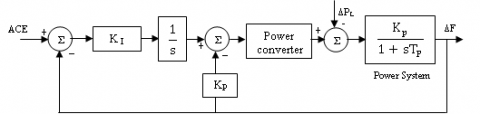Figure 2. Block diagram for PDF control

3.1 Flower Pollination Algorithm

Yang  developed a recently putforth an algorithm which essentially obtained on the principle of flower pollination process of flowering plant. The main itention of the pollination process is fittest for surviving and to produce optimal number of trees to make them more survival. Relying upon this optimization behavior of flower pollination process an optimization technique is developed which is known as Flower Pollination Algorithm (FPA). Pollination is the process through which there is the reproduction with the help of flowers. Pollination process is of mainly two types. By nature it is abiotic and biotic. In abiotic pollination process is obtained without any pollinator rather it is done by wind and water. But in the case of biotic, pollination process is done with the help of pollinators like some insects and animals. Insects like honey bees while transitting through many flowers increase the pollen revolution and thus exploit the reproduction due to flower constancy. For pollinators flower constancy is helpful, because due to this nectar supply is obtained at very less memory and at low cost of learning. Potentially it produces more new flower species in place of concentrating towards un avoidable things. So flower constancy relates to low functional cost with surety of nectar intake. Pollination process is obtained by self-pollination and cross-pollination. In self-pollination process of one flower fertilization or pollen of same flower whereas cross-pollination process is obtained by pollen of different flower species. Insects like birds, bats and flies can fly long distances easily so they produce pollination in long distances which is known as biotic cross pollination also termed as global pollination. By taking the difference and equality of different flowers, constancy attribute may be used for incremental step in pollination process. The pollination process is the inspiration of flower pollination algorithm . Apart from pollination process some characteristics like pollination constancy and pollinator behavior, which are main cause for creation of some rules in FPA. (i) The universal pollination procedure are biotic and cross pollination through which the pollen transfer the pollinators to perform the levy flight, (ii) Local pollination is outlook as abiotic and self pollination. (iii) Reproduction chance is considered as flower constancy which is proportional to the matching of the two flowers in concerned and (iv) The switching probability guarded both the local and global pollination $p \in\left[\begin{array}{ll}0, & 1\end{array}\right]$. This process guarantees pollination and reproduction of the fittest solution represented as g*. The flower constancy can be represented as:

$x_{i}^{t+1}=x_{i}^{t}+L\left(x_{i}^{t}-g_{*}\right)$            (9)

where $x_{i}^{t}$ is the pollen i or solution vector $x_{i}$ at iteration t, and $g_{*}$ is the current best solution found among all solutions at the current generation/iteration. The parameter L is the strength of the pollination, which essentially is a step size. Since insects may move over a long distance with various distance steps, which is mimicked by levy distribution of flight [12-14] and mathematically represented as

$L \sim \frac{\lambda \Gamma(\lambda) \sin \left(\frac{\pi \lambda}{2}\right)}{\pi} \frac{1}{s^{1+\lambda}}\left(s \ll s_{0}>0\right)$                 (10)

where $\Gamma(\lambda)$ is the standard gamma function, and this distribution is valid for large steps s > 0. The local pollination and flower constancy can be represented as

$x_{i}^{t+1}=x_{i}^{t}+\varepsilon\left(x_{i}^{t}-x_{k}^{t}\right)$                     (11)

where $\chi_{j}^{t}$ and $x_{k}^{t}$ represent pollen from different flowers of the same species of plant. Thus, mimic the flower constancy in a limited neighborhood. The switch probability or proximity probability is used to switch between common global pollination to intensive local pollination. The adequacy of the FPA can be attributed to the accompanying reasons:

(I) Insect pollinators can go in long separations which empower the FPA to maintain a strategic distance from neighborhood scene to look in a huge space (investigations). (ii) The FPA guarantees that comparative types of the blooms are reliably picked which ensure quick union to the ideal arrangement (misuse). The proposed blossom fertilization calculation for settling AGC application.

1. Step 1: Initialize the objective function as given in the equation (8)

2. Step 2: Initialize a population of x = (x1, x2 ...xNF) flowers/pollen gametes with the population size of NF x N. Where NF is the number of flowers 30 and N is the dimension size depends on the number of controller gain values for each area in the two area system. In this study N is equal to four because PI controller is used to in each area (KP1, KI1 KP2, KI2) and calculate the Fitness for each solutions.

3. Step 3: Find the optimal solution to the initial population and define a switch possibility $\mathrm{p} \in[0,1]$ and define a stopping criterion (a fixed number of generations/iterations)

4. Step 4: while (t <Maximum Generation) for i = 1: n (all n flowers in the population) if rand < p, Draw a (d-dimensional) step vector L which follows a Levy distribution Global pollination has been done using equation (9). Else draw from a uniform distribution in [0, 1]. Randomly choose jth and kth flower among all the solutions and do local pollination through equation (11), end if

5. Step 5: Calculate new solutions using the objective function. If new solutions are superior, update them in the population, end for

6. Step 6: Find the current best solution based on the objective fitness value, end while.

4. Function Hydrogen Energy Storage (HES) in AGC Loop

The hydrogen energy storage is a process of splitting the water into hydrogen and oxygen by giving the direct current to the electrodes in an electrolysis cells; hydrogen is then compressed into a tank, so that energy can be stored in the form of hydrogen gas, then the hydrogen energy is converted back to electricity by fuel cells and few other equipments. Fuel cells are devices normally used to convert hydrogen energy back to electricity for easy operation and higher efficiency compared with other devices used to convert the hydrogen to electricity. The important elements of a HES unit involves with an electrolyzer unit which converts electrical energy to hydrogen by the decomposition of water molecules as shown in Figure 3.

## 3.pngFigure 3. Block Schematic of the hydrogen Energy storage unit

The gain value of the function in 'S' domain of the Aqua Electrolyzer can be expressed as first order lag:

$G_{A E}(s)=\frac{K_{A E}}{1+s T_{A E}}$                      (12)

The gain value of the function in 'S' domain of Fuel Cell (FC) can be given by a simple linear equation as

$G_{F C}(s)=\frac{K_{F C}}{1+S T_{F C}}$                (13)

The overall gain value of the function in 'S' domain of Hydrogen Energy storage unit has can be

$G_{H E S}(s)=\frac{K_{H E S}}{1+S T_{H E S}}=\frac{K_{A E}}{1+s T_{A F}} * \frac{K_{F C}}{1+s T_{F C}}$            (14)

5. Power System Restoration

The system restoration methodologies are found firmly identified with the systems characteristics. After surfed and analysed the system conditions and outage characteristics, system restoration planners or dispatchers will select the Power System Restoration Indices (PSRI) which were obtained based on system dynamic performances and the remedial measures to be taken can be decided. In this work, two-area thermal-thermal and thermal-diesel interconnected power system in a restructured environment are considered when the system is operating in a typical condition with Gencos units in operation and are one or more Gencos unit outage in any area.

From these Restoration Indices the restorative estimates like the magnitude of control input, rate of change of cont rol input required can be considered. The various power system restoration indices $\left(P S R I_{1}, P S R I_{2}, P S R I_{3}\right.$ and $\left.P S R I_{4}\right)$ are calculated as follows.

Step 1: The Power System Restoration Index 1 $\left(P S R I_{1}\right)$ is attained from the proportion between the settling time of the control input deviation $\Delta P_{c 1}\left(\tau_{s 1}\right)$ response of area 1 and power system time constant $\left(T_{p 1}\right)$ of area 1

$P S R I_{1}=\frac{\Delta P_{C 1}\left(\tau_{S 1}\right)}{T_{p 1}}$                   (15)

Step 2: The Power System Restoration Index 2 $\left(P S R I_{2}\right)$ is attained from the proportion between the settling time of the control input deviation $\Delta P_{c 2}\left(\tau_{s 2}\right)$ response of area 2 and power system time constant $\left(T_{p 2}\right)$ of area 2

$P S R I_{2}=\frac{\Delta P_{c 2}\left(\tau_{s 2}\right)}{T_{p 2}}$                    (16)

Step 3: The Power System Restoration Index 3 $\left(P S R I_{3}\right)$ is attained from the peak value of the control input deviation $\Delta P_{c 1}\left(\tau_{p}\right)$ response of area 1 with respect to the final value $\Delta P_{c 1}\left(\tau_{s}\right)$

$P S R I_{3}=\Delta P_{c 1}\left(\tau_{p}\right)-\Delta P_{c 1}\left(\tau_{s}\right)$                (17)

Step 4: The Power System Restoration Index 4 $\left(P S R I_{4}\right)$ is attained from the peak value of the control input deviation $\Delta P_{c 2}\left(\tau_{p}\right)$ response of area 1 with respect to the final value $\Delta P_{c 1}\left(\tau_{s}\right)$

$P S R I_{4}=\Delta P_{c 2}\left(\tau_{p}\right)-\Delta P_{c 2}\left(\tau_{s}\right)$                 (18)

6. Simulation Results and Observations

The proposed controller is tuned using Flower Pollination Algorithm (FPA) and implemented two-area thermal reheat interconnected deregulated power system for different transactions. In this test system consists of two Gencos and Discos in each area. The nominal constraints are given in Appendix. The best possible solution of control inputs are taken for optimization problem and the objective function in Eqn (8) is derived using the frequency deviations of control areas and tie- line power changes. The active power model of HES unit is installed in area1 to inspect its effect on the power system performance. The optimum PDF controller gain values for the test systems without and with HES unit are tuned for various case studies are listed in the Table 1 and Table 2 respectively.

Scenario 1: Poolco based transaction

In this scenario, Gencos take part only in the load following control of their areas. It is supposed to be imagnine that a huge step load 0.15 p.u MW is demanded by each Disco in area 1. Assume that a case of Poolco based contracts between Dicos and available Gencos is simulated based on the following Disco Participation Matrix (DPM) referring to Eq (1) is considered as

$D P M_{1}=\left[\begin{array}{llll}0.5 & 0.5 & 0.0 & 0.0 \\ 0.5 & 0.5 & 0.0 & 0.0 \\ 0.0 & 0.0 & 0.0 & 0.0 \\ 0.0 & 0.0 & 0.0 & 0.0\end{array}\right]$           (19)

Table 1. Optimal PDF controller gain values using FPA for two-area reheat thermal- thermal power system with corresponding Load demand change

 Two-area thermal system PDF controller gain of area 1 PDF controller gain of area 2 Load demand in pu.MW un contracted load demand pu.MW KP KI KP KI Disco1 Disco2 Disco3 Disco4 area1 area 2 Case 1 0.392 0.543 0.236 0.227 0.15 0.15 0.0 0.0 0.0 0.0 Case 2 0.411 0.447 0.304 0.234 0.15 0.15 0.0 0.0 0.1 0.0 Case 3 0.474 0.508 0.342 0.248 0.15 0.15 0.0 0.0 0.0 0.1 Case 4 0.493 0.452 0.354 0.251 0.15 0.15 0.0 0.0 0.1 0.1 Case 5 0.437 0.493 0.361 0.253 0.25 0.05 0.25 0.05 0.0 0.0 Case 6 0.403 0.575 0.219 0.311 0.25 0.05 0.25 0.05 0.1 0.0 Case 7 0.436 0.591 0.221 0.328 0.25 0.05 0.25 0.05 0.0 0.1 Case 8 0.441 0.597 0.337 0.267 0.25 0.05 0.25 0.05 0.1 0.1 Case 9 0.457 0.585 0.353 0.343 0.20 0.10 0.20 0.10 0.0 0.0 Case 10 0.464 0.667 0.283 0.364 0.20 0.10 0.20 0.10 0.1 0.0 Case 11 0.484 0.631 0.374 0.286 0.20 0.10 0.20 0.10 0.0 0.1 Case 12 0.501 0.688 0.366 0.323 0.20 0.10 0.20 0.10 0.1 0.1

Table 2. Optimal PDF controller gain values using FPA for two-area reheat thermal- thermal power system with HES unit for corresponding Load demand change

 Two-area thermal system PDF controller gain of area 1 PDF controller gain of area 2 Load demand in pu.MW un contracted load demand pu.MW KP KI KP KI Disco1 Disco2 Disco3 Disco4 area1 area 2 Case 1 0.316 0.624 0.198 0.289 0.15 0.15 0.0 0.0 0.0 0.0 Case 2 0.334 0.512 0.223 0.312 0.15 0.15 0.0 0.0 0.1 0.0 Case 3 0.405 0.562 0.264 0.318 0.15 0.15 0.0 0.0 0.0 0.1 Case 4 0.412 0.508 0.308 0.322 0.15 0.15 0.0 0.0 0.1 0.1 Case 5 0.396 0.581 0.312 0.327 0.25 0.05 0.25 0.05 0.0 0.0 Case 6 0.314 0.631 0.191 0.381 0.25 0.05 0.25 0.05 0.1 0.0 Case 7 0.354 0.684 0.194 0.393 0.25 0.05 0.25 0.05 0.0 0.1 Case 8 0.362 0.662 0.284 0.324 0.25 0.05 0.25 0.05 0.1 0.1 Case 9 0.395 0.691 0.297 0.426 0.20 0.10 0.20 0.10 0.0 0.0 Case 10 0.398 0.728 0.214 0.437 0.20 0.10 0.20 0.10 0.1 0.0 Case 11 0.408 0.742 0.322 0.361 0.20 0.10 0.20 0.10 0.0 0.1 Case 12 0.412 0.753 0.326 0.402 0.20 0.10 0.20 0.10 0.1 0.1

Table 3. PSRI for two-area interconnected power systemwithout and with HES unit using FPA based PDF controller

 Load demand change PSRI for thermal-thermal power system without HES unit PSRI for thermal-thermal power system with HES unit PSR PSR PSR PSR $\int P_{c}$ PSR PSR PSR PSR $\int P_{c}$ Case 1 0.975 0.886 0.133 0.027 1.056 0.801 0.704 0.082 0.006 0.978 Case 2 1.326 1.025 0.297 0.045 3.262 0.806 0.882 0.114 0.010 2.872 Case 3 1.185 1.322 0.224 0.067 0.782 0.914 0.911 0.118 0.013 0.578 Case 4 1.461 1.375 0.302 0.085 3.947 1.025 1.061 0.219 0.039 3.457 Case 5 0.926 0.875 0.148 0.095 1.261 0.795 0.698 0.098 0.051 1.157 Case 6 1.325 1.025 0.326 0.101 3.499 0.904 0.939 0.186 0.071 3.078 Case 7 1.234 1.327 0.215 0.184 1.031 0.831 1.021 0.152 0.141 0.989 Case 8 1.376 1.345 0.341 0.196 3.269 1.002 1.085 0.245 0.153 3.108 Case 9 1.134 1.517 0.346 0.298 1.103 1.001 1.237 0.301 0.235 1.008 Case 10 1.524 1.524 0.383 0.341 3.194 1.081 1.343 0.310 0.301 3.087 Case 11 1.345 1.623 0.432 0.496 1.894 1.001 1.418 0.371 0.417 1.798 Case 12 1.627 1.735 0.457 0.512 3.271 1.427 1.551 0.382 0.474 3.127

## 4a.png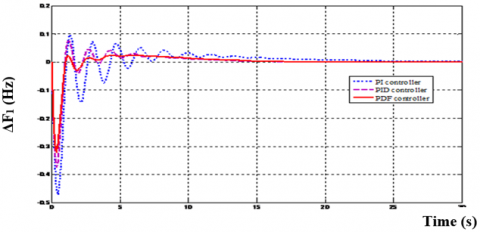(a) ΔF1 (Hz) Vs Time(s)

## 4b.png(b) ΔF2 (Hz) Vs Time (s)

## 4c.png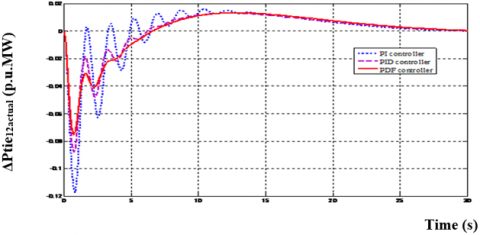(c) ΔPtie12, actual (p.u.MW) Vs Time (s)

## 4d.png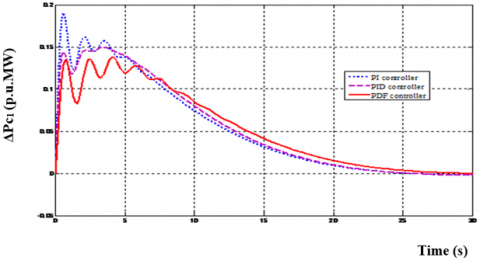(d) ΔPc1 (p.u.MW) Vs Time (s)

## 4e.png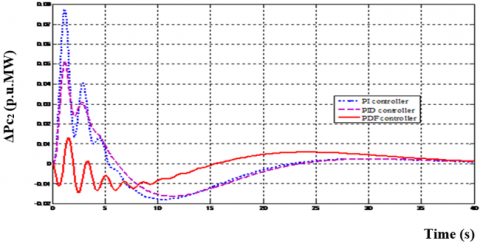(e) ΔPc2 (p.u.MW) Vs Time (s)

Figure 4. Dynamic responses of the frequency deviations, tie- line power deviations, and Control input deviations for a two area thermal-thermal system using different controllers (case-1)

## 5a.png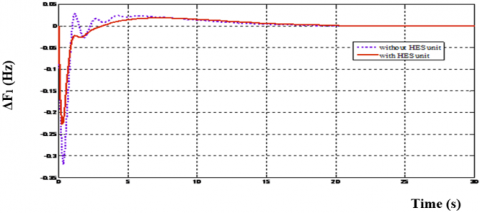(a) ΔF1 (Hz) Vs Time(s)

## 5b.png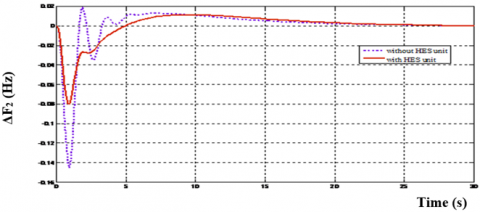(b) ΔF2 (Hz) Vs Time (s)

## 5c.png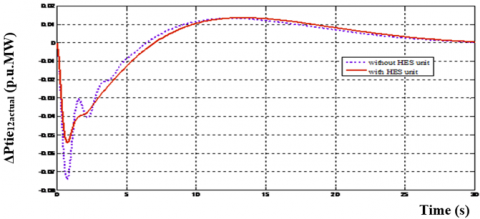(c)ΔPtie12, actual (p.u.MW) Vs Time (s)

## 5d.png(d) ΔPc1 (p.u.MW) Vs Time (s)

## 5e.png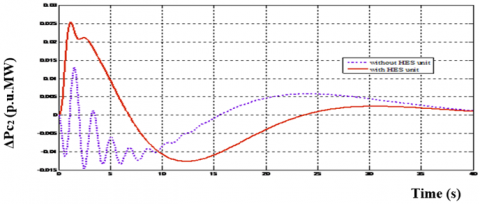(e) ΔPc2 (p.u.MW) Vs Time (s)

Figure 5. Dynamic responses of the frequency deviations, tie- line power deviations, and Control input deviations for a two area thermal-thermall system without and with HES unit using PDF controllers (case-1)

Disco1 and Disco2 demand identically from their local Gencos, viz., Genco1 and Genco2. Therefore, cpf11 = cpf12 = 0.5 and cpf21 = cpf22 = 0.5. It may happen that a Disco infringe a contract by demanding more power than that particular point in the contract and this surplus power is not contracted to any of the Gencos. This uncontracted power must be supplied by the Gencos in the same area to the Disco. It is represented as a local load of the area but not as the contract demand. Consider scenario-1 again with a alteration that Disco demands as given in Table 1 and Table 2. The designed PDF controllers are implemented in a proposed power system for dissimilar types of transactions and matched with PI and PID controller. The dynamic ouput response of the reheat thermal system with different types controller is shown in Figure 4. From Figure 4, it can be seen that the proposed PDF controller show better performance as compared with PI and PID controller. The active power model of HES unit is installed in area1 to inspect its effect on the power system performance. The relative transient performances of two-area thermal-thermal deregulated power system with HES units using PDF controller are shown in Figure 5 and it can observed that the oscillations in area frequencies and tie-line power deviation have flattened to a significable extent as matched upto that of the system without HES units. The corresponding PSRI are calculated using Eqns (15)-(18) from dynamic responses of control input deviations of each area for various types of probable transactions of the proposed test system is shown in Table 3 (case 1- 4). From the Table 3, it is also additionally seen that the PSRI computed for the test system with HES unit demonstrates that more advanced control for a superior restoration of the power system output responses and to ensure enhanced PSRI in order to provide good margin of stability than that of the system without HES unit.

Scenario 2: Bilateral based transaction

In this juncture, all the Discos have contract with the Gencos and the following Disco Participation Matrix (DPM) referring to Eq (1) is considered as

$D P M=\left[\begin{array}{llll}0.4 & 0.2 & 0.4 & 0.5 \\ 0.1 & 0.3 & 0.2 & 0.3 \\ 0.3 & 0.2 & 0.2 & 0.1 \\ 0.2 & 0.3 & 0.2 & 0.2\end{array}\right]$             (20)

In this case, the Disco1, Disco2, Disco3 and Disco4, demands 0.25 pu.MW, 0.05 pu.MW, 0.25 pu.MW and 0.05 pu.MW from Gencos as described by cpf in the DPM matrix and each Gencos participates in AGC as elaborately given by the following ACE participation factor apf11 = apf12 = 0.5 and apf21 = apf22 = 0.5. The corresponding PSRI are calculated using Eq (15)-(18) from dynamic responses of control input deviations of the proposed test system are shown in Table 3 (case 5- 8). Looking to the context again, the normal operating condition of the test systems few other case studies like outage generating unit in any area and uncontracted power demand in any area during outage is taken into account.

In this study Genco2 in area 1 is outage and uncontracted power demand in any area and Disco Participation Matrix Eq (20) is considered. The optimum PDF controller gain values for two test system without and with HES unit are tuned for various case studies are tabulated in the Table 1and 2 respectively (case 9-12). These PDF controllers are implemented in a proposed test system for different type of transactions. From simulation results the dynamic responses of control input deviations of the proposed test system the corresponding PSRI using Eq (15)-(18) and tabulated in Table 3 (case 9- 12).  From these Restoration Indices the restorative measures like the magnitude of control input, rate of change of control input required can be considered. From the simulated results is shown in Table 3, it is keenly observed that the restoration procedure with the HES unit ensures not only robust operation but provides a good margin of stability compared with that of a system without HES unit.

6.1. Power System Restoration Assessment

The main heart in this paper PSRI are useful for system planners for restoration arrangement in advance.

(i) If $1.0 \leq P S R I_{1}, P S R I_{2} \leq 2.0$, then the system subject to a large steady error for step load changes. The integral control action is required based on the execution criteria. The integral controller gain of each control area has to be increased causing the speed changer valve to open up generally. Thus the speed- changer position attains a consistent value only when the frequency error is reduced to zero.

(ii) If $P S R I_{1}, P S R I_{2} \geq 2.0$, then the system required more amount of distributed generation requirement is needed and the FACTS devices are needed to improvement tie-line power oscillations.

(iii) If $0.05 \leq P S R I_{3}, P S R I_{4} \leq 0.1$, then the system required the consistent in of frequency oscillations in an interconnected power system. The traditional / conventional load-frequency controller may no longer be able to attenuate the large frequency oscillation due to the slow response of the governor for erratic load variations. Thus the progressing change in power system rrangements and their operating conditions might lead to unfavourable operation of relays. So that in deregulated system, regulation and load following are the two frequency-related ancillary services required for balancing the changeable loads with matching generation. In cases where a vivid decline in frequency occurs during the restoration process, it is necessary to reduce the amount of load that are connected, which can be accomplished by the application of under load shedding scheme.

(iv) If $P S R I_{3}, P S R I_{4} \leq 0.2$, then the system is exposable and the system becomes unstable and may result to ouatages. To regain the system as quickly as possible, especially for a massive system, partition or sliding door system into islands are necessary. Islands are reharmonise after restoration of each island. Majority of the actions involved in this restoration process are start up of black start units, cranking of non-black start units, restoration of islands, and synchronization of islands.

7. Conclusion

The PDF controllers are structured using FPA technique and the efficacy of the proposed controller is tested in a two-area thermal-thermal deregulated power system for a wide range of load demands and perturbation under dissimilar operating conditions. The various simulated ouputs show that the FPA based PDF controller’s performance is swift, more accurate and better than the simulated results with PI and PID controllers. HES unit is included in area 1 in order to improve the system performance. It is seen clearly that in all the cases (poolco based, bilateral based and contract violation based) the variation of frequency becomes zero in the steady state with less setting time because of the coordinated application of HES units which assures the prime requirement of AGC. Moreover, the proposed PID controller with AGC system has HES units that demonstrates better performance to ensure the improvement of PSRI in order to offer lower restoration time with improved system reliability.

Acknowledgment

The authors wish to thank the authorities of Annamalai University, Annamalainagar, Tamilnadu, India for the facilities provided to prepare this paper.

Appendix

Data for the interconnected two- area interconnected Power System 

Rating of each area = 2000 MW, Base power = 2000 MVA, fo = 60 Hz, R1 = R2 = R3 = R4 = 2.4 Hz / p.u.MW, Tg1 = Tg2 = Tg3 = Tg4 = 0.08 s, Tr1 = Tr2 = Tr1 = Tr2 = 10 s, Tt1 = Tt2 = Tt3 = Tt4 = 0.3 s, Kp1 = Kp2 = 120Hz/p.u.MW, Tp1 = Tp2 = 20 s, b1 = b2 = 0.425 p.u.MW / Hz, Kr1 = Kr2 = Kr3 = Kr4 = 0.5, THES = 0.04 sec, $2\pi {{T}_{12}}$= 0.545 p.u.MW / Hz, a12 = -1.

References

 Pandey, S.K., Mohanty, S.R., Kishor, N. (2013). A literature survey on load–frequency control for conventional and distribution generation power systems. Renewable and Sustainable Energy Reviews, 25: 318-334. https://doi.org/10.1016/j.rser.2013.04.029

 Pujara, S.M., Kotwal, C.D. (2016). An inclusive review on load frequency control in deregulated market. International Journal on Electrical Engineering and Informatics, 8(3): 595. https://doi.org/10.15676/ijeei.2016.8.3.9

 Pappachen, A., Fathima, A.P. (2017). Critical research areas on load frequency control issues in a deregulated power system: A state-of-the-art-of-review. Renewable and Sustainable Energy Reviews, 72: 163-177. https://doi.org/10.1016/j.rser.2017.01.053

 Donde, V., Pai, M.A., Hiskens, I.A. (2001). Simulation and optimization in an AGC system after deregulation. IEEE transactions on power systems, 16(3): 481-489. https://doi.org/10.1109/59.932285

 Nain, P., Parmar, K.S., Singh, A.K. (2013). Automatic generation control of an interconnected power system before and after deregulation. International Journal of Computer Applications, 61(15): 11-16.

 Saikia, L.C., Nanda, J., Mishra, S. (2011). Performance comparison of several classical controllers in AGC for multi-area interconnected thermal system. International Journal of Electrical Power & Energy Systems, 33(3): 394-401. https://doi.org/10.1016/j.ijepes.2010.08.036

 Shayeghi, H.A.S.H., Shayanfar, H.A., Jalili, A. (2009). Load frequency control strategies: A state-of-the-art survey for the researcher. Energy Conversion and management, 50(2): 344-353. https://doi.org/10.1016/j.enconman.2008.09.014

 Sahu, R.K., Gorripotu, T.S., Panda, S. (2016). Automatic generation control of multi-area power systems with diverse energy sources using teaching learning based optimization algorithm. Engineering Science and Technology, an International Journal, 19(1): 113-134. https://doi.org/10.1016/j.jestch.2015.07.011

 Chidambaram, I.A., Paramasivam, B. (2013). Optimized load-frequency simulation in restructured power system with redox flow batteries and interline power flow controller. International Journal of Electrical Power & Energy Systems, 50: 9-24. https://doi.org/10.1016/j.ijepes.2013.02.004

 Erol, O.K., Eksin, I. (2006). A new optimization method: big bang–big crunch. Advances in Engineering Software, 37(2): 106-111. https://doi.org/10.1016/j.advengsoft.2005.04.005

 Kumar, N., Kumar, V., Tyagi, B. (2016). Optimization of PID parameters using BBBC for a multiarea AGC scheme in a deregulated power system. Turkish Journal of Electrical Engineering & Computer Sciences, 24(5): 4105-4116.

 Yang, X.S. (2012). Flower pollination algorithm for global optimization. In International conference on unconventional computing and natural computation, 240-249. https://doi.org/10.1007/978-3-642-32894-7_27

 Yang, X.S., Karamanoglu, M., He, X. (2014). Flower pollination algorithm: a novel approach for multiobjective optimization. Engineering optimization, 46(9): 1222-1237. https://doi.org/10.1080/0305215X.2013.832237

 Madasu, S.D., Kumar, M.S., Singh, A.K. (2018). A flower pollination algorithm based automatic generation control of interconnected power system. Ain Shams Engineering Journal, 9(4): 1215-1224. https://doi.org/10.1016/j.asej.2016.06.003

 Shankar, R., Chatterjee, K., Bhushan, R. (2016). Impact of energy storage system on load frequency control for diverse sources of interconnected power system in deregulated power environment. International Journal of Electrical Power & Energy Systems, 79: 11-26. https://doi.org/10.1016/j.ijepes.2015.12.029

 Chatterjee, K. (2011). Effect of battery energy storage system on load frequency control under deregulation. International Journal of Emerging Electric Power Systems, 12(3): 12-17, https://doi.org/10.2202/1553-779X.2700

 Liu, Y., Fan, R., Terzija, V. (2016). Power system restoration: a literature review from 2006 to 2016. Journal of Modern Power Systems and Clean Energy, 4(3): 332-341. https://doi.org/10.1007/s40565-016-0219-2

 Phelan, R.M., Johnson, S.H. (1977). Automatic control systems. Cornell University Press, Ithaca, New York, 1977.